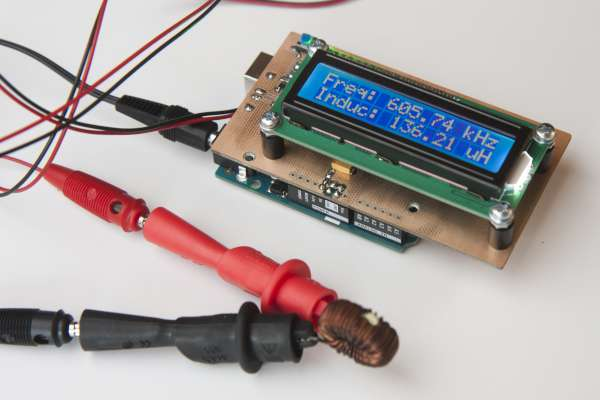# Arduino-based Inductance Meter

I’ve just finished a little Arduino project. It’s a shield for the Arduino Uno that lets you measure inductance. This is a functionality that I found missing in just about any digital multi meter. Yes, there are specialized LCR meters that let you measure inductance but they typically won’t measure voltages or currents. So I had to build my inductance meter myself.

The basic design is really simple. It a colpitts oscillator (http://en.wikipedia.org/wiki/Colpitts_oscillator) with the coil missing. You use the test leads to connect it to a coil which will make it resonate. The Arduino then measures the frequency at which the oscillator is resonating and calculates the inductance. The capacitors are part of the shield so the capacity is known.There is 1uH of inductance included on the schield which is placed in series with the coil to be measured. This serves two purposes: The oscillator can resonate when you short-circuit the test leads. When you then press the push button on the shield, the software will use the current measurement as new calibration. It also puts an upper limit on the resonance frequency. This ensures that the software the rest of the circuit can keep up with the oscillator.

As can be seen from the schematic, the oscillator uses two 1nF capacitors in series. Together with the 1uH inductance, this limits the frequency to about 7.1MHz. In practice, it oscillates at around 5.4MHz when the test leads are short-circuited.

The oscillator output is followed by a comparator turning the sine wave of the oscillator into a square wave. I’ve used an inexpensive but fast Microchip MCP6561R. It has a maximum propagation delay of 80ns which allows it to keep up at the maximum frequency.

For more detail: Arduino-based Inductance Meter

#### This Post / Project can also be found using search terms:

• how to program inductance measurement using arduino led shield